# Interpolating Scanner Circuit

J. Donald Tillman
January 1999

## Abstract

This is an original design for an interpolating scanner, a circuit with a number of signal inputs, a control voltage input and a signal output. The output effectively selects between the inputs, fading from one to the next, as the control voltage rises.

Prior scanner circuits include:

• the electromechanical scanner used on Hammond Organs (ref) which uses capacitive coupling between spinning metal plates.
• Juergen Haible's interpolating scanner which uses opamp circuits generating triangular windows to drive current controlled amplifiers.
• A two-channel panner circuit might be considered a degenerate form of a scanner.  The data sheet for the Precision Monolithics, Inc. SSM-2024 current-controlled amp IC shows a stereo panner, they call it an "Exponential Cross-Fade Controller", where two CCAs are controlled from a PNP differentialamp. The circuit is pretty straightforward and I'm sure similiar circuits have been used before.

The circuit presented here is inspired by and similiar to JH's scanner except that drive circuit is an unusual extrapolation of the differential amplifier topology used in the PMI panner. This approach has some advantages:

• a smoother transition between inputs
• the total drive current (the sum of all the drive currents) is from a constant current source assuring that the sum of the individual gains is held constant
• low parts count
• control of the transition shape

The main disadvantage is that it requires transistor and diode matching for best performance (although it seems to work pretty well for unmatched devices).

The design is extendible to greater or fewer inputs.

## Interface Requirements

For this particular design I'm using the following interface specs:

8 signal inputs.
Signal inputs are -10 dBu, 0.7 volts p-p, 50K ohms, DC to high audio (roughly 20KHz).
Signal output is -10 dBu, 0.7 volts p-p, low impedance, DC to high audio.
Control voltage input is between -2.5 and +2.5 volts, 50K ohms, DC to high audio.

## Scanner Design

Figure 1 below shows a block diagram of the Interpolating Scanner. There is a Current Controlled Amplifer (CCA) of traditional design for each signal input. Each CCA interfaces appropriately to the input signal, has a current source output, and a gain (transconductance) that is directly proportional to its control current input. The CCA outputs are summed by shorting them directly together. A buffer amplifier stage provides the appropriate output level and impedance.Figure 1. Block diagram of the interpolating scanner.

The CCAs are transconductance amplifiers; the input is a voltage, the output is a current, the gain is linearly proportional to the control current, and in this case the control current is expected to be from 0.0 to 2.0 mA. More on this later.

Figure 2 below shows the details of the Scanner Drive circuit.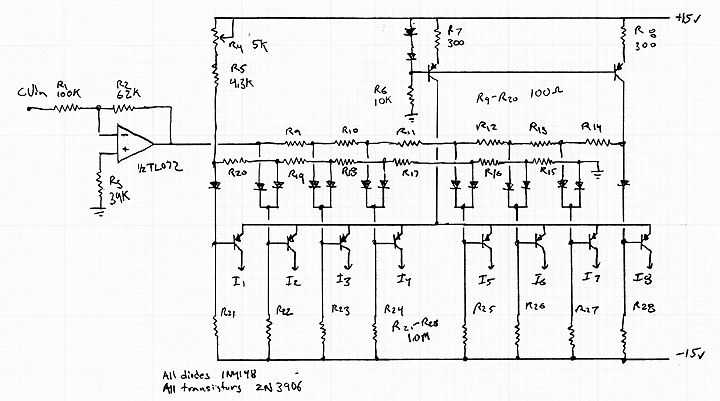Figure 2. Scanner drive schematic.

Figure 3 below shows schematics of the CCAs and the buffer amp.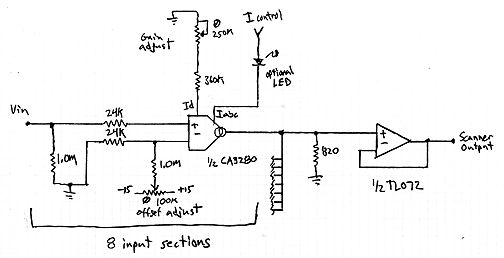Figure 3. Current Controlled Amplifier and Buffer Amp.

## Scanner Drive Theory of Operation

In the schematic above, the opamp provides a flexible interface to the outside world (you can add multiple inputs here as well as manual controls), scales the control voltage slightly, and supplies a low impedance source of the control voltage to the rest of the circuit.

The issue in extending a diff amp to more than two inputs is to not only gradually turn one transistor off and another on, but also turn that second one off and the next one on in the same manner.

Resistors R9 through R14 provide 190mV voltage steps up from the control voltage and resistors R15 through R20 provide 190mV voltages steps up from ground. The diodes select the higher of the two values.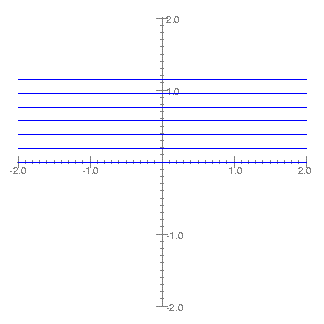Here are three plots I drew to show how this works.

The X axis is the voltage tapped off of the opamp in volts and the Y axis is the various base voltages in volts. For clarity, the base voltages here are before the base diodes so offset everything down by one diode voltage drop to get the actual base voltages.

In the first plot we see the constant voltages presented by the resistor string on the right. These are 190 mV apart.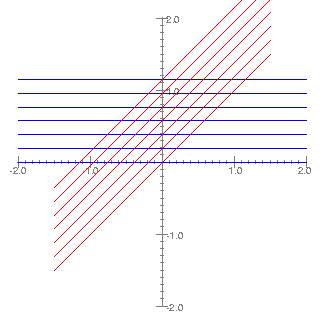In the second plot we also see a string of input signal voltages, presented by the resistor string on the left. These voltages are also 190mV apart.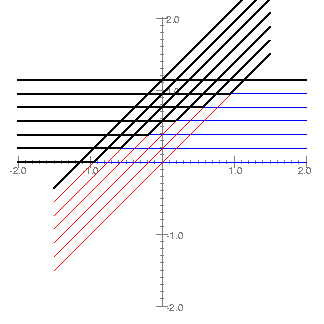In the third plot we see the effect of the diodes, selecting the most positive of each of the voltage pairs. Again, for clarity this diagram shows voltages before the diode voltage drop. Note that the extreme outputs are not paired. Also note that the voltage between crossover points is two voltage steps.

## Shape Control

There is some control over the shape of the interpolation curve. The circuit presented above is optimized for a smooth and complete transition from one stage to the next, but by varying the control voltage swing and the difference between the voltage steps you can customize the curves.

Here are three plots from a SPICE simulation of the scanner drive circuit. All have the input control voltage on the X axis, 1 volt per division, and the individual output currents on the Y axis, 0.5mA per division, with EIA colors representing output currents I1 through I8.The first plot is with double the step voltage and shows very sharp transistions. It is the scanner drive circuit above with the following values changed:

step voltage = 380mV
R2 = 33 k
R4,5 = 3.3k
R8 = 150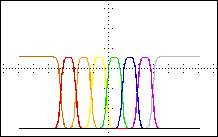The second plot is the schematic above.

step voltage = 190mV
R4,5 = 6.8k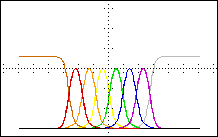The third plot is with roughly half the step voltage. Note that at the extremes of the input range all of current from the current source is channeled through I1 or I8, but the intermediate outputs have so much spread that they steal from their neighbor's peak currents as the sum current stays constant.

step voltage = 90mV
R2 = 33k
R4,5 = 16k
R8 = 620

A potential strategy for an optimal shape would be to select step voltage value where the I2 through I7 peak currents are 95% of the I1 or I8 shelf currents; 95% percent being a somewhat arbitrarily choosen value near unity. It is possible to calculate an approximation to the appropriate step voltage by solving the equation of a differential amp in this situation:

Vstep = 2 * 0.026 * ln( 2 * (Rp / (1-Rp)))

Where Vstep is the step voltage and R is the ratio of the peak current of one of the intermediate outputs (I2 through I7) to the shelf current of the outside stages (I1, I8). For Rp = 0.95, Vstep is 190 mV, and this is the value used in the schematic.

## Matching Issues

Do the transistors in this circuit need to be matched? Close matching is not required, and depending upon the application, none may be necessary. For most electronic music applications I would suggest the minimal matching procedure: check the forward voltage of the diodes and the emitter-base junctions of the transistors at some low current, say 100 uA, and throw out the ones with extreme numbers.

I built a breadboard version of this circuit without matching the transistors, and differences in the curves of the individual outputs were visible, but not bad. As I threw out extreme values the curves matched up better.

## Current Controlled Amplifiers

The current controlled amplifiers are based on the CA3280 operational transconductance amplifier (OTA). This model was chosen because

• it has very low noise
• it has linearizing input diodes to minimize distortion
• it has a linear control current to gain relationship
• the control current is referenced to near the negative supply voltage
(makes the drive circuit a little easier to design)
• it will take a higher control current than most
(this allows LEDs to be inserted in the current control signals)

In this design the input diodes are biased to 30uA which will set the amp's input impedance to roughly 2.0 kohms.  With the two 24 kohm input resistors this presents at 50 kohm input resistance and an input attnuation of 0.04.  Standard -10 dBu audio signals run 0.7 v p-p and will be brought down to 27mV p-p at the OTA input terminals.

The scanner drive is designed for a maximum control current of 2.0 mA, high enough to directly power an low-current LED indicator in series with the control current input (thanks to JH for this suggestion) as well as supplying plenty of gain.

The transconductance of the CA3280 OTA is:

Gm = 15 * Iabc
So the common OTA load resistance is choosen for unity gain:
Rload = 1 / ([Rd / Rin(total)]) * Gm(max))
which comes to 820 ohms.

Control of the transition shape with a front panel knob. This might be especially useful if the scanner was going to be used as a synthesizer module. I would replace R4,5 with a current source just like on the other side and run them together with a dual pot which would also adjust the control voltage gain.

Stereo. Simply double up the CCAs and buffer amps; run both sets off of the same scanner drive.

## Applications

The primary application I had in mind for this device was for a modern analog implementation of the Hammond Flying Chorus. I'm currently writing an article describing it.

As JH has suggested, an interpolating scanner module for a modular synthesizer can be very useful both for creating waveforms and control voltages.

## Status

I have built breadboards of several versions of the scanner drive circuit. I have not built the CCAs or a whole scanner unit yet. Currently I'm working on a three input scanner project.

## References

Hammond Organ Service Manual, http://theatreorgans.com/hammond/faq/a-100/a-100.html

Juergen Haible, Interpolating Scanner, March 1998.

Precision Monolithics Inc., SSM-2024 Quad Current Controlled Amplifier Data Sheet, November 1989, from PMI Data Book, Volume 10, Analog Integrated Circuits, 1990.